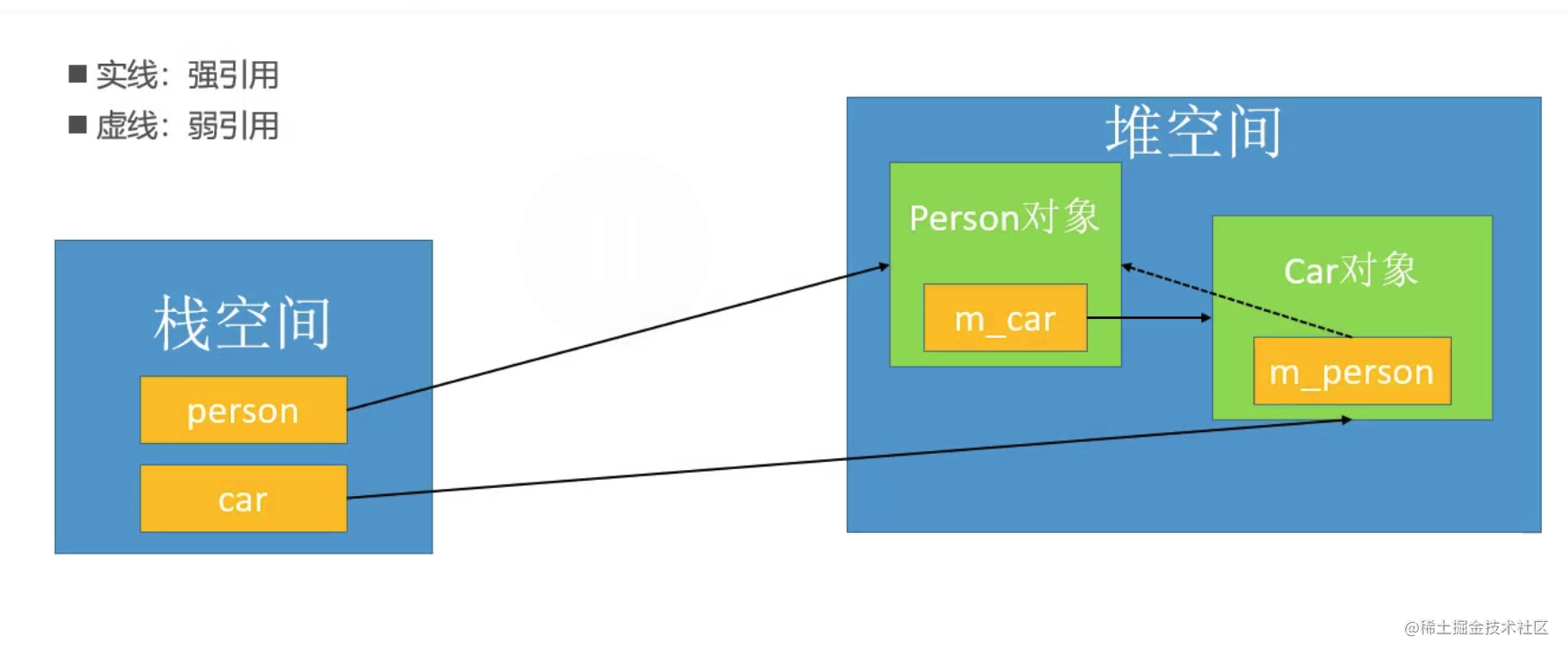# C++ Day14-其他语法 C 智能指针

##### 1. C++ 中传统指针存在什么问题？
• ①需要手动管理内存
• ②容易发生内存泄漏（忘记释放、出现异常等）
• ③释放后产生野指针
##### 2. 为了解决传统指针的痛点，引入了什么指针？只能指针的格式怎么样？智能指针指向堆对象还是栈对象？
• 引入了智能指针（Smart Pointer）：auto_ptr(有缺陷，不能用于数组，已废弃)、shared_ptr、unique_ptr
• 智能指针指向`堆对象`
• 如果使用指针指针指向`栈对象`，因为栈对象本身出了作用于就会被释放，智能指针会导致再次释放，会有 `double free` 问题
``````int main() {
try {
// 智能指针 p 指向了 堆空间的person对象(千万不要用智能指针指向栈空间对象，栈空间)
shared_ptr<Person> p(new Person());
p->run();
throw "异常了";
} catch (...) {
cout << "异常了" << endl;
}
// 即使抛出异常，`~Person() ` 还是得到即使释放
return 0;
}

##### 3. 如何实现一个简单的自定义智能指针？
``````template <typename T>
class SmartPtr {
T *m_obj;
public:
SmartPtr(T *data): m_obj(data) {}

~SmartPtr() {
if (m_obj == nullptr) return;
delete m_obj;
}
// 重载运算符 -> 才能正常调用 m_obj 的方法
T * operator->() {
return m_obj;
}
};

using namespace std;

int main() {
cout << 1 << endl;
{
SmartPtr<Person> sp = SmartPtr<Person>(new Person());
sp->run();
}
cout << 3 << endl;
return 0;
}

##### 4. shared_ptr 对比 auto_ptr 有什么优点？
• 多个 shared_ptr 可以指向同一个对象，当最后一个 shared_ptr 在作用域内结束时，对象才会被自动释放
• 可以通过一个已存在的智能指针初始化一个新的智能指针
• shared_ptr 和 OC 里面的普通指针简直一模一样
##### 5. 简述 shared_ptr 的原理
• 一个 `shared_ptr` 会对一个对象产生`强引用（Strong reference）`
• 每个对象都有个与之对应的`强引用计数`，记录着当前对象被多少个 shared_ptr 强引用在着，可以通过 shared_ptr 的 use_count 函数获得强引用计数
• 当有一个新的 shared_ptr 指向对象的时候，对象的强引用计数就会 +1
• 当有一个 shared_ptr 销毁时（比如作用域结束），对象的强引用计数就会 -1
• 当一个对象的强引用计数为 0 时（没有任何 share_ptr 指向对象时），对象就会自动销毁（析构函数调用）
``````int main() {
shared_ptr<Person> p1;
{
shared_ptr<Person> p2 = shared_ptr<Person>(new Person());
cout << p2.use_count() << endl; // 1
p1 = p2;
cout << p1.use_count() << endl; // 2
shared_ptr<Person> p3 = p2;
cout << p1.use_count() << endl; // 3
}
cout << p1.use_count() << endl; // 1
return 0;
}

##### 6. 下面代码，运行会出问题吗？
``````int main() {
Person *p = new Person();

{
shared_ptr<Person> p1(p);
}

{
shared_ptr<Person> p2(p);
}

return 0;
}

• 会出现多次释放 p 的情况，会崩溃
##### 7. 智能指针如何出现循环引用？怎么解决？• `weak_ptr` 会对一个对象产生`弱引用`
• `weak_ptr` 可以解决 shared_ptr 的循环引用问题
##### 8. unique_ptr（没啥用）
• 保证只有一个智能指针指向对象